# Angle Modulation

The other type of modulation in continuous-wave modulation is the Angle Modulation. Angle Modulation is the process in which the frequency or the phase of the carrier varies according to the message signal. This is further divided into frequency and phase modulation.

• Frequency Modulation is the process of varying the frequency of the carrier signal linearly with the message signal.

• Phase Modulation is the process of varying the phase of the carrier signal linearly with the message signal.

Let us now discuss these topics in greater detail.

## Frequency Modulation

In amplitude modulation, the amplitude of the carrier varies. But in Frequency Modulation (FM), the frequency of the carrier signal varies in accordance with the instantaneous amplitude of the modulating signal.

The amplitude and the phase of the carrier signal remains constant whereas the frequency of the carrier changes. This can be better understood by observing the following figures.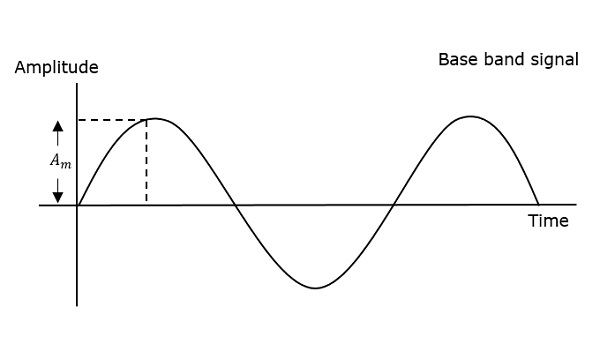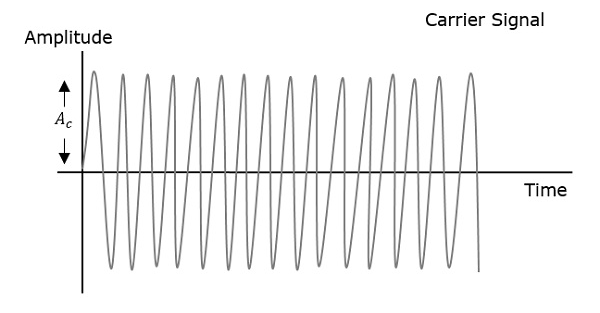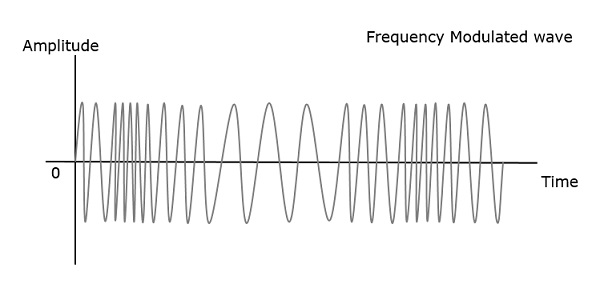The frequency of the modulated wave remains constant as the carrier wave frequency when the message signal is at zero. The frequency increases when the message signal reaches its maximum amplitude.

Which means, with the increase in amplitude of the modulating or message signal, the carrier frequency increases. Likewise, with the decrease in the amplitude of the modulating signal, the frequency also decreases.

### Mathematical Representation

Let the carrier frequency be fc

The frequency at maximum amplitude of the message signal = fc + Δf

The frequency at minimum amplitude of the message signal = fcΔf

The difference between FM modulated frequency and normal frequency is termed as Frequency Deviation and is denoted by Δf.

The deviation of the frequency of the carrier signal from high to low or low to high can be termed as the Carrier Swing.

Carrier Swing = 2 × frequency deviation

= 2 × Δf

### Equation for FM WAVE

The equation for FM wave is −

$$s(t) = A_ccos[W_ct + 2\pi k_fm(t)]$$

Where,

Ac = the amplitude of the carrier

wc = angular frequency of the carrier = 2πfc

m(t) = message signal

FM can be divided into Narrowband FM and Wideband FM.

### Narrowband FM

The features of Narrowband FM are as follows −

• This frequency modulation has a small bandwidth.

• The modulation index is small.

• Its spectrum consists of carrier, USB, and LSB.

• This is used in mobile communications such as police wireless, ambulances, taxicabs, etc.

### Wideband FM

The features of Wideband FM are as follows −

• This frequency modulation has infinite bandwidth.

• The modulation index is large, i.e., higher than 1.

• Its spectrum consists of a carrier and infinite number of sidebands, which are located around it.

• This is used in entertainment broadcasting applications such as FM radio, TV, etc.

## Phase Modulation

In frequency modulation, the frequency of the carrier varies. But in Phase Modulation (PM), the phase of the carrier signal varies in accordance with the instantaneous amplitude of the modulating signal.

The amplitude and the frequency of the carrier signal remains constant whereas the phase of the carrier changes. This can be better understood by observing the following figures.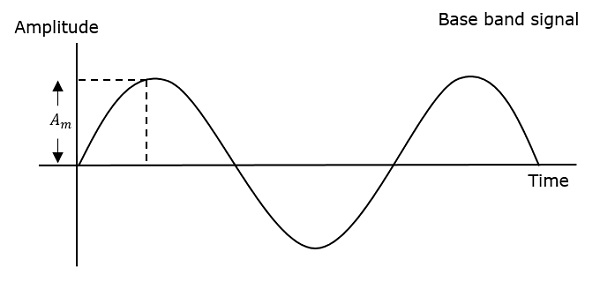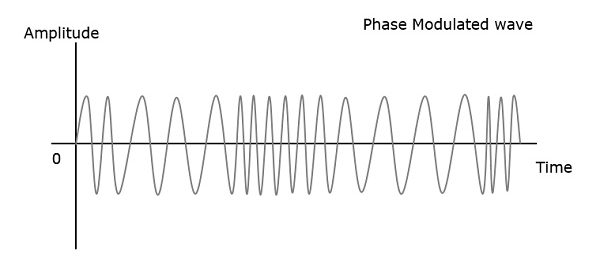The phase of the modulated wave has got infinite points where the phase shift in a wave can take place. The instantaneous amplitude of the modulating signal, changes the phase of the carrier. When the amplitude is positive, the phase changes in one direction and if the amplitude is negative, the phase changes in the opposite direction.

## Relation between PM and FM

The change in phase, changes the frequency of the modulated wave. The frequency of the wave also changes the phase of the wave. Though they are related, their relationship is not linear. Phase modulation is an indirect method of producing FM. The amount of frequency shift, produced by a phase modulator increases with the modulating frequency. An audio equalizer is employed to compensate this.

### Equation for PM Wave

The equation for PM wave is −

$$s(t) = A_ccos[W_ct + k_pm(t)]$$

Where,

Ac = the amplitude of the carrier

wc = angular frequency of the carrier = 2πfc

m(t) = message signal

Phase modulation is used in mobile communication systems, while frequency modulation is used mainly for FM broadcasting.